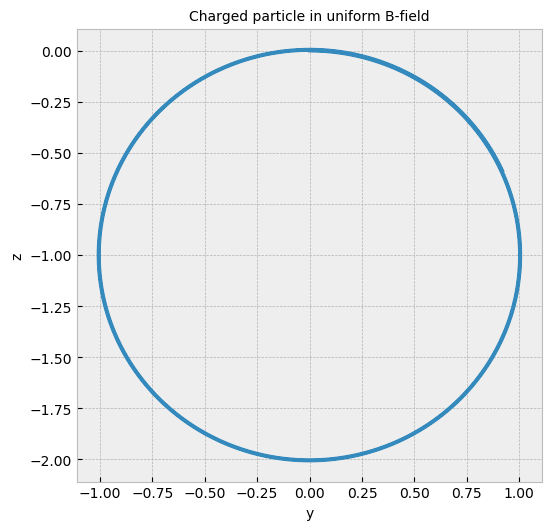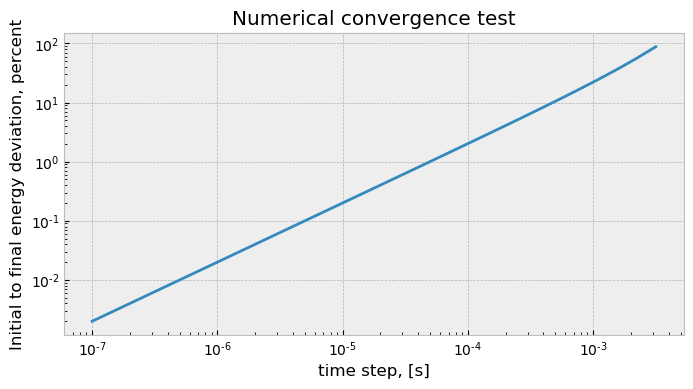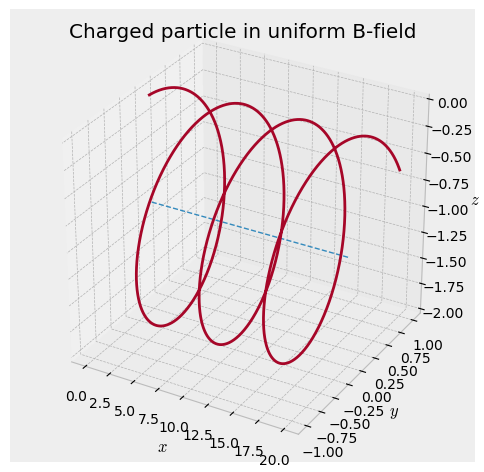# Uniform Magnetic Field¶

### Examples - Electromagnetism¶

Last edited: September 1st 2019

## Introduction¶

A charged particle moving in a magnetic field will experience a magnetic force governed by Lorentz force law. This force is always perpendicular to the direction of motion of the particle. If the particle moves in uniform magnetic field and the velocity of the particle is normal to the direction of the field, the particle end up moving in a circle. Now, if the direction of the field is not normal to the velocity, the particle starts moving in a helix, unless its motion is completely parallel to the field in which the there is no Lorentz force and the particle moves in a perfectly straight line. In this notebook we will try to simulate the two former scenarios by numerically integrate the particle's equations of motion using the Euler method.

## Theory¶

### The Lorentz force law¶

A particle with charge $Q$ and mass $m_p$ moving with a velocity $\mathbf{v}$ in a magnetic field $\mathbf{B}$ experiences a force

\begin{equation} \mathbf{F}_{mag} = Q(\mathbf{v} \times \mathbf{B}) \label{Lorentz} \end{equation}

known as the Lorentz force . Here, bold symbols represent vectors while normal symbols represent scalars.

### Discretisation¶

The Lorentz force can be written as a second order ordinary differential equation (ODE)

\begin{equation} m_p\frac{d^2\mathbf{x}}{dt^2} = Q\big(\frac{d\mathbf{x}}{dt} \times \mathbf{B}\big), \label{LorentzODE} \end{equation}

where the velocity components are written as the time derivative of the positional components $v_i = dx_i/dt$. The left hand side of the equation is of course Newton's second law, where $d^2\mathbf{x}/dt^2$ is the acceleration of the particle written as the second derivative of the position with respect to time. By decomposing the vectors, \eqref{LorentzODE} can be reduced into three sets of two first order ODE's.

\begin{equation} \begin{aligned} &\frac{dx}{dt} = v_x\\[4pt] &\frac{dv_x}{dt} = \frac{Q}{m_{p}}(v_yB_z - v_zB_y)\\[10pt] &\frac{dy}{dt} = v_y\\[4pt] &\frac{dv_y}{dt}= \frac{Q}{m_{p}}(v_zB_x - v_xB_z)\\[10pt] &\frac{dz}{dt} = v_z\\[4pt] &\frac{dv_z}{dt} = \frac{Q}{m_{p}}(v_xB_y - v_yB_x) . \end{aligned} \label{LorentzComponents} \end{equation}

Using a numerical method such as the Euler method or Runge-Kutta fourth order, we can find approximate solutions for the ODE using a specified time step, $\Delta t$, and the motion of a charged particle in a magnetic field can be found.

## Numerical validity¶

### Conservation of energy¶

Since the Lorentz force is a cross product between the particle's velocity and the magnetic field, its direction is always perpendicular to the velocity of the particle. Consequently, the Lorentz force does no work on the particle, and the particle's kinetic energy is conserved. A test for numerical validity is then to check the deviation in initial and final kinetic energy.

As stated in the introduction, a charged particle will move in a circle if it has a velocity component perpendicular to an external magnetic field. This is called Larmor gyration, and the gyroradius (or Larmor radius), the radius of the circle, is given by 

\begin{equation} r_L = \frac{mv_{\perp}}{|Q| B} , \label{larmorRadius} \end{equation}

where $v_{\perp}$ is the velocity of the particle perpendicular to the magnetic field with magnitude $B$.

## Implementation¶

In :
# First, import all necessary library packages
import numpy as np              # For math and array manipulation
import matplotlib.pyplot as plt # For plotting
from mpl_toolkits.mplot3d import Axes3D # For 3D-plotting
plt.style.use("bmh")            # Set a particular style on the plots
# "Magic" command to adapt matplotlib plots to jupyter notebook
%matplotlib inline
figsize = (6, 6)                # Tuple tho hold figure size of plots
dpi = 100                       # Dots per inch on plots (resolution)


A charged particle moving through a uniform magnetic field can easily be simulated using the Euler method with the equations \eqref{LorentzComponents}. Click here for a notebook on Euler's method. Below we define a function for the right hand side of the equations in \eqref{LorentzComponents}, as well as a function for one explicit Euler step, given the current position and velocity.

In :
def RHS(M, RHSargs):
""" Returns the right hand side of the sets of ordinary
differential equations.
Arguments:
M         1x6 array containing the position and
velocity of the particle in cartesian
coordinates.
RHSargs   1x5 array containing the arguments/variables
of the right hand side of the Lorentz force.
"""
x, y, z, vx, vy, vz = M
Q, mass, Bx, By, Bz = RHSargs
ddt_vx = Q/mass*(vy*Bz - vz*By)
ddt_vy = Q/mass*(vz*Bx - vx*Bz)
ddt_vz = Q/mass*(vx*By - vy*Bx)
ddt_x = vx
ddt_y = vy
ddt_z = vz
return ddt_x, ddt_y, ddt_z, ddt_vx, ddt_vy, ddt_vz

def eulerStep(M, dt, RHS, RHSargs):
""" Performs one step of the Euler method."""
x, y, z, vx, vy, vz = M # Extract positional and velocity components from matrix
dx, dy, dz, dvx, dvy, dvz = RHS(M, RHSargs) # Calculate right hand side of ODE's
# Increment each component using Euler's method
x = x + dx*dt
y = y + dy*dt
z = z + dz*dt
vx = vx + dvx*dt
vy = vy + dvy*dt
vz = vz + dvz*dt
return x, y, z, vx, vy, vz


For now, we want the particle to move in a uniform magnetic field with an initial velocity perpendicular to the field direction.

In :
Q = 1                   # Particle charge
mass = 1                # Particle mass
Bx = 10                 # Magnetic field x-component magnitude
By = 0                  # Magnetic field y-component magnitude
Bz = 0                  # Magnetic field z-component magnitude
h = 1e-4                # Time step for numerical integration
tMax = 2                # Total duration of integration
n = int(tMax/h)         # Number of datapoints

# Array of arguments (parameters) to the RHS function
RHSargs = np.array([Q, mass, Bx, By, Bz])

# Initialising data matrix
M = np.zeros((6, n))

# Initial position and velocity components
x0 = 0    # x-component magnitude of positional vector
y0 = 0    # y-component magnitude of positional vector
z0 = 0    # z-component magnitude of positional vector
vx0 = 0   # x-component magnitude of velocity vector
vy0 = 10  # y-component magnitude of velocity vector
vz0 = 0   # z-component magnitude of velocity vector
M[:, 0] = np.array([x0, y0, z0, vx0, vy0, vz0]) # Storing the components in the data matrix

# The matrix M will have the following form when completly filled with data:
#
# M[ROW, COLUMN]
#
#           n COLUMNS
#    -----------------------------------
# 6 | x0   x1   x2   ...  x_n-2   x_n-1
#   | y0   y1   y2   ...  y_n-1   y_n-1
# R | z0   z1   z2   ...  z_n-1   z_n-1
# O | vx0  vx1  vx2  ...  vx_n-1  vx_n-1
# W | vy0  vy1  vy2  ...  vy_n-1  vy_n-1
# S | vz0  vz1  vz2  ...  vz_n-1  vz_n-1
#
# Writing ":" in M[ROWS, COLUMNS] such as M[:, 0] returns an array containing
# the first column. M[0, :] returns the first row
#

# Integrate particle trajectory
for i in range(n-1):
M[:, i+1] = eulerStep(M[:, i], h, RHS, RHSargs)

# Calculating deviation in kinetic energy
# Check out scipy.org for a description of the numpy-function numpy.linalg.norm(),
# here we use it to calculate the absolute value of the velocity by squaring its components.
Ke_initial = 0.5*mass*np.absolute(np.linalg.norm(M[3:6, 0], axis=0))**2 # mv^2/2 (formula for kinetic energy)
Ke_final = 0.5*mass*np.absolute(np.linalg.norm(M[3:6, -1], axis=0))**2
energyDeviation = np.abs(Ke_final - Ke_initial)/Ke_initial*100
print("Initial to final energy deviation:\t\t%.3f percent"%(energyDeviation))

# Plot path
plt.figure(figsize=(6, 6), dpi=dpi)
plt.plot(M, M)
plt.xlabel('y', size=10)
plt.ylabel('z', size=10)
plt.title("Charged particle in uniform B-field", size=10)

plt.show()

Initial to final energy deviation:		2.020 percentThe particle moves in a circle in the $yz$-plane, as expected by equation \eqref{Lorentz}. Our parameters yields the Larmor radius $r_g = 1$ from equation \eqref{larmorRadius}. By a quick look at the axis above it seems accurate.

Concerning conservation of energy, we see that the kinetic energy deviate with $2\%$ from its initial value, indicating that the time step was roughly appropriate for this integration interval. However, if integrating over a longer period (larger tmax), this deviation might grow to large because of the accumulating numerical error in each Euler step, and the numerical validity weakens. It may be wise to run a convergence test using different time steps and comparing the total error.

In :
# Creating an array of timeSteps with logarithmic spacing
timeSteps = np.logspace(-7, -2.5, 30)
# Array to hold deviations for each time step
deviations = np.zeros((len(timeSteps)))

Ke_initial = 0.5*mass*np.absolute(np.linalg.norm(M[3:6, 0], axis=0))**2

for k in range(len(timeSteps)):
h = timeSteps[k]
n = int(tMax/h)   # Number of datapoints
# Initialising data matrix
M = np.zeros((6, n))
M[:, 0] = np.array([x0, y0, z0, vx0, vy0, vz0]) # Storing the components in the data matrix

# Integrate particle trajectory
for i in range(n-1):
M[:, i+1] = eulerStep(M[:, i], h, RHS, RHSargs)

# Calculating deviation in kinetic energy
Ke_final = 0.5*mass*np.absolute(np.linalg.norm(M[3:6, -1], axis=0))**2
energyDeviation = np.abs(Ke_final - Ke_initial)/Ke_initial*100
deviations[k] = energyDeviation

plt.figure(figsize=(8, 4), dpi=dpi)
plt.loglog(timeSteps, deviations)
plt.xlabel('time step, [s]')
plt.ylabel('Initial to final energy deviation, percent')
plt.title('Numerical convergence test')
plt.show()From the plot above we see that the error in kinetic velocity is first order in the time step $\Delta t$. This result also indicate that a time step below $10^{-5}\mathrm{s}$ is sufficient to get a deviation below $0.1\%$.

Now, how does the particle's trajectory look if the initial velocity is in both $x$ and $y$ direction?

In :
vx0 = 10             # Resetting vx0 to 10
h = 1e-5
n = int(tMax/h)      # Recalculating number of datapoints
M = np.zeros((6, n)) # Re-initialising data matrix
M[:, 0] = np.array([x0, y0, z0, vx0, vy0, vz0])

for i in range(n-1):
M[:, i+1] = eulerStep(M[:, i], h, RHS, RHSargs)
# Calculating deviation in kinetic energy
Ke_initial = 0.5*mass*np.absolute(np.linalg.norm(M[3:6, 0], axis=0))**2 # mv^2/2 (formula for kinetic energy)
Ke_final = 0.5*mass*np.absolute(np.linalg.norm(M[3:6, -1], axis=0))**2
energyDeviation = np.abs(Ke_final - Ke_initial)/Ke_initial*100
print("Initial to final energy deviation:\t\t%.3f percent"%(energyDeviation))

fig = plt.figure(figsize=(6,6), dpi=100)
# Plotting guiding line
ax.plot(M, M*0, np.ones(len(M))*-1, '--', linewidth=1)
# Plotting particle path
ax.plot(M, M, M)
ax.set_xlabel(r"$x$")
ax.set_ylabel(r"$y$")
ax.set_zlabel(r"$z$")
ax.set_title("Charged particle in uniform B-field")

plt.show()

Initial to final energy deviation:		0.100 percentWe get a helical motion! The velocity in $x$-direction is unchanged as consequence of the cross-product in the Lorentz force law. The blue line is the movement of the centre of Larmor gyration. This is called the guiding centre.